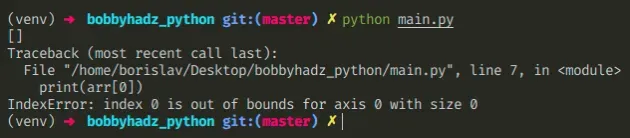# IndexError: index 0 is out of bounds for axis 0 with size 0Fri Apr 22 20222 min readPhoto by Ann Danilina

## IndexError: index 0 is out of bounds for axis 0 with size 0#

The Python "IndexError: index 0 is out of bounds for axis 0 with size 0" occurs when we try to access the first item in the first dimension of an empty numpy array. To solve the error, use a `try/except` block or check if the array's size is not equal to `0` before accessing it at an index.Here is an example of how the error occurs.

main.py
```Copied!```import numpy as np

arr = np.array([])

print(arr.shape)  # 👉️ (0,)
print(arr.size)  # 👉️ 0

# ⛔️ IndexError: index 0 is out of bounds for axis 0 with size 0
print(arr)
``````

We tried to access the first element in an empty array which caused the error.

You can use the `shape` property to print the shape of the array or the `size` property to print its size (the number of elements in the array).

Note that numpy uses zero-based indexing - the first element in an array has an index of `0`.

One way to solve the error is to check if the array's size is greater than `0` before accessing its first item.

main.py
```Copied!```import numpy as np

arr = np.array([])

if arr.size > 0:
print(arr)
else:
print('array has a size of 0')
``````

The `if` block is only ran if the size of the array is greater than `0`, otherwise the `else` block runs.

Alternatively, you can use a `try/except` statement to handle the error.

main.py
```Copied!```import numpy as np

arr = np.array([])

try:
print(arr)
except IndexError:
print('array is empty')
``````

We tried accessing the array element at index `0` which raised an `IndexError` exception.

You can handle the error or use the `pass` keyword in the `except` block.

When declaring a numpy array, make sure it has the correct dimensions.

main.py
```Copied!```import numpy as np

arr_1 = np.array([1, 2, 3])

print(arr_1.shape)  # 👉️ (3,)
print(arr_1.size)  # 👉️ 3
print(arr_1)  # 👉️ 1

# 👇️ array with 2 rows and 3 columns
# [[0 0 0]
#  [0 0 0]]
arr_2 = np.zeros((2, 3), dtype=int) #
print(arr_2.shape)  # (2, 3)

print(arr_2)  # 👉️ [0 0 0]
print(arr_2)  # 👉️ 0
print(arr_2.size) # 👉️ 6
``````

Axis `0` is the first dimension of an array and the `size` property can be used to get the number of elements in the array.

For two-dimensional arrays, the first set of square brackets is used to specify the index of the selected row, and the second set is used to specify the column (the value inside a row).

Make sure you aren't creating an array with 0 rows and N columns.

main.py
```Copied!```arr_2 = np.zeros((0, 3), dtype=int)
print(arr_2)  # 👉️ []
print(arr_2.shape)  # (0, 3)

# ⛔️ IndexError: index 0 is out of bounds for axis 0 with size 0
print(arr_2)
``````

The array above has a size `0` for its first dimension, so try to access any element at index would cause an `IndexError`.

Use the search field on my Home Page to filter through my more than 1,000 articles.